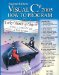# Wrap-Up

### Answers to Self Review Exercises

 27.1 a) enumerator (or foreach statement). b) ArrayList. c) indexer. d) double. e) Synchronized. f) MoveNext. g) InvalidOperationException. 27.2 a) True. b) False. The class must also implement property Current. c) True. d) False. An ArrayList stores only objects. Autoboxing occurs when adding a value type to the ArrayList. You can prevent boxing by instead using generic class List with a value type. e) True. f) False. A Hashtable cannot contain duplicate keys. g) True. h) False. Dictionary is a class; IDictionary is an interface. i) False. An enumerator cannot be used to change the values of elements. j) False. With hashing, as the load factor increases, there are fewer available slots relative to the total number of slots, so the chance of selecting an occupied slot (a collision) with a hashing operation increases.

### Exercises

 27.3 Define each of the following terms: ICollection Array IList load factor collision spacetime trade-off in hashing Hashtable 27.4 Explain briefly the operation of each of the following methods of class ArrayList: Add Insert Remove Clear RemoveAt Contains IndexOf Count Capacity 27.5 Explain why inserting additional elements into an ArrayList object whose current size is less than its capacity is a relatively fast operation and why inserting additional elements into an ArrayList object whose current size is at capacity is a relatively slow operation. 27.6 In our implementation of a stack in Fig. 25.13, we were able to quickly extend a linked list to create class StackInheritance. The .NET Framework designers chose not to use inheritance to create their Stack class. What are the negative aspects of inheritance, particularly for class Stack? 27.7 Briefly answer the following questions: What happens when you add a simple type (e.g., double) value to a non-generic collection? Can you print all the elements in an IEnumerable object without explicitly using an enumerator? If yes, how? 27.8 Explain briefly the operation of each of the following enumerator-related methods: GetEnumerator Current MoveNext 27.9 Explain briefly the operation of each of the following methods and properties of class Hashtable: Add Keys Values ContainsKey 27.1 Determine whether each of the following statements is true or false. If false, explain why. Elements in an array must be sorted in ascending order before a BinarySearch may be performed. Method First gets the first node in a LinkedList. Class Array provides static method Sort for sorting array elements. 27.11 Write an application that reads in a series of first names and stores them in a LinkedList. Do not store duplicate names. Allow the user to search for a first name. 27.12 Modify the application in Fig. 27.8 to count the number of occurrences of each letter rather than of each word. For example, the string "HELLO THERE" contains two Hs, three Es, two Ls, one O, one T and one R. Display the results. 27.13 Use a SortedDictionary to create a reusable class for choosing from some of the predefined colors in class Color (in the System.Drawing namespace). The names of the colors should be used as keys, and the predefined Color objects should be used as values. Place this class in a class library that can be referenced from any C# application. Use your new class in a Windows application that allows the user to select a color and then changes the background color of the Form. 27.14 Write an application that determines and prints the number of duplicate words in a sentence. Treat uppercase and lowercase letters the same. Ignore punctuation. 27.15 Recall from Fig. 27.2 that class List is the generic equivalent of class ArrayList. Write an application that inserts 25 random integers from 0 to 100 in order into an object of class List. The application should calculate the sum of the elements and the floating-point average of the elements. 27.16 Write an application that creates a LinkedList object of 10 characters, then creates a second list object containing a copy of the first list, but in reverse order. 27.17 Write an application that takes a whole-number input from a user and determines whether it is prime. If the number is not prime, display the unique prime factors of the number. Remember that a prime number's factors are only 1 and the prime number itself. Every number that is not prime has a unique prime factorization. For example, consider the number 54. The prime factors of 54 are 2, 3, 3 and 3. When the values are multiplied together, the result is 54. For the number 54, the prime factors output should be 2 and 3. Use generic SortedDictionarys as part of your solution by recording the factors as the keys and using the Keys property to enumerate the factors. 27.18 In Exercise 24.7, you performed a bucket sort of ints by using a two-dimensional array, where each row of the array represented a bucket. By instead using a dynamically expanding data structure to represent each bucket, you do not have to write code that keeps track of the number of ints in each bucket. Rewrite your solution to use a one-dimensional array of LinkedList< int > buckets.Visual C# 2005 How to Program (2nd Edition)
ISBN: 0131525239
EAN: 2147483647
Year: 2004
Pages: 600

Similar book on Amazon